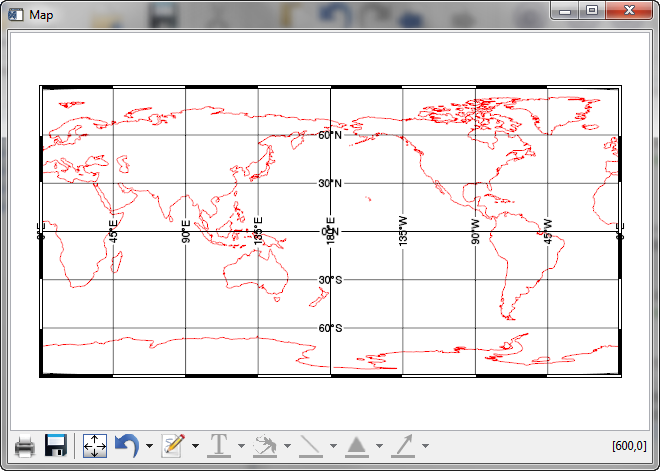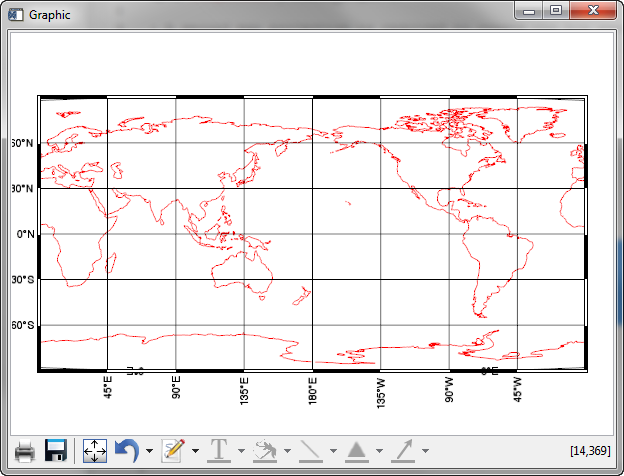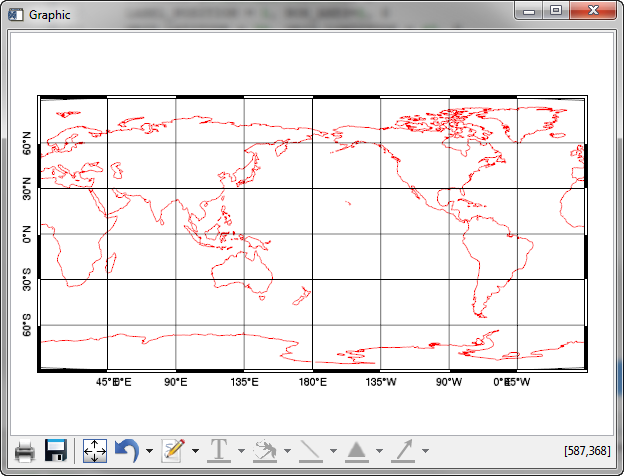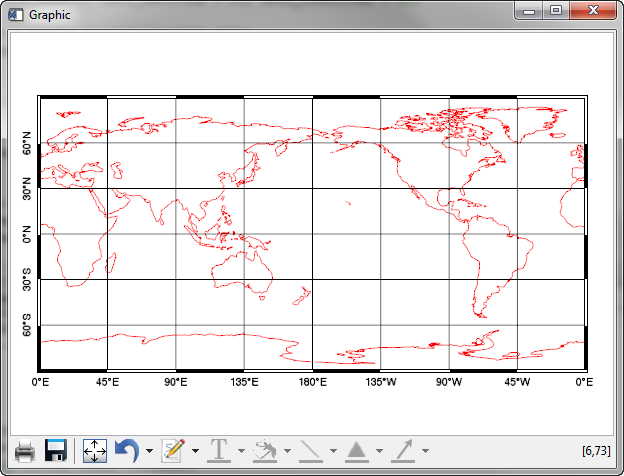# Box Axes with the Map FunctionQUESTION: I think everyone understands what I mean when I say I want box axes on a map projection. But, when I set the Box_Axes keyword on the IDL 8 Map function, I don't get anything like what I expect. For example, this code produces the results shown in the figure below.

```   mp = Map('Equirectangular', CENTER_LONGITUDE=180, \$
BOX_AXES=1, GRID_LATITUDE = 30, GRID_LONGITUDE = 45)
c = MapContinents(Color=!Color.red)
```The map projection resulting from setting the Box_Axes keyword to the Map function.

Clearly, the box axes are there, but the axis labeling is daft. How can I fix this?ANSWER: Yes, this is not a very intuitive result, I agree.

It turns out that if you want to have a map with box axes labelled in the normal way, you have a bit of work to do. First of all, you have to move the locations where the labeling occurs on the map to the edge of the map. You can either set the labeling the the left and bottom of the map by setting the Label_Position keyword to 0, or you can set the labeling the the top and right of the map by setting the Label_Position keyword to 1. Note that you cannot label all four sides of the map as is normally done with box axes with a single map projection command. (More on this below.)

To move the labels to the left and bottom, you can type this.

```   mp = Map('Equirectangular', CENTER_LONGITUDE=180, \$
BOX_AXES=1, GRID_LATITUDE = 30, GRID_LONGITUDE = 45, \$
LABEL_POSITION=0)
c = MapContinents(Color=!Color.red)
```The box axes labels moved to the right and bottom of the map.

The problem here, of course, is that the map labels are orientated perpendicular to the axes, while they should be oriented parallel to the axes for box axes. We can solve this problem by re-orienting the axis labels. The code will look like this.

```   mp = Map('Equirectangular', CENTER_LONGITUDE=180, \$
BOX_AXES=1, GRID_LATITUDE = 30, GRID_LONGITUDE = 45, \$
LABEL_POSITION=0)
mp['Latitudes'].label_angle=90
mp['Longitudes'].label_angle=0
c = MapContinents(Color=!Color.red)
```The map box axes labels are orientated in the proper way.

You will notice in the figure above, however, some problem with the axes labels at 45 degrees east and west. It turns out these are extraneous labels that are put on the map as a result of a bug (we believe!) in the Map function in IDL 8.2.1. The extraneous labels appear as a result of the latitude range going from its default values of -90 to 90 degrees. If we use the Limit keyword to the Map function, and restrict the latitude limits so they are not exactly -90 and 90 degrees, we can eliminate these extraneous labels.

```   mp = Map('Equirectangular', CENTER_LONGITUDE=180, \$
BOX_AXES=1, GRID_LATITUDE = 30, GRID_LONGITUDE = 45, \$
LABEL_POSITION=0, LIMIT=[-89.99, 0, 89.99, 360])
mp['Latitudes'].label_angle=90
mp['Longitudes'].label_angle=0
c = MapContinents(Color=!Color.red)
```Extraneous labels are removed by restricting the latitude limit.

To label the top and right axes, you need to create another set of axes labels. The code to do so looks like this.

```   mp = Map('Equirectangular', CENTER_LONGITUDE=180, \$
BOX_AXES=1, GRID_LATITUDE = 30, GRID_LONGITUDE = 45, \$
LABEL_POSITION=0, LIMIT=[-89.99, 0, 89.99, 360])
mp['Latitudes'].label_angle=90
mp['Longitudes'].label_angle=0
grid = MapGrid( \$
LONGITUDE_MIN=0, LONGITUDE_MAX=360, \$
LATITUDE_MIN=-90, LATITUDE_MAX=90, \$
GRID_LONGITUDE=45, GRID_LATITUDE=30, \$
LABEL_POSITION=1)
FOREACH g,grid.latitudes DO g.label_angle=270
FOREACH g,grid.longitudes DO g.label_angle=0
c = MapContinents(Color=!Color.red)
```A normal looking Box Axes map projection with the Map function.